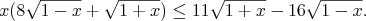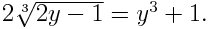# Main list of math "coffin" problems

## The problems

• Problem 1. A solution is available at http://arxiv.org/abs/1110.1556

Solve the following inequality for positive x:• Problem 2.

In a given tetrahedron, the sums of the lengths of pairs of opposite edges are the same for all such pairs. Prove that the sums of pairs of opposite dihedral angles are the same.

• Problem 3.

A bisector of a trihedral angle is defined as the locus of points that are equidistant from its (three) edges. Find a necessary and sufficient condition for two bisectors of a tetrahedron to intersect.

• Problem 4.

Given a fixed volume, find a tetrahedron with that volume that maximizes the radius of its inscribed sphere.

• Problem 5.

Denote by M the set of numbers of the type A + B√2, where A and B are integers. Prove that any neighborhood of a real number contains an infinite number of elements of the set M.

Here is the idea, submitted by Simon Elimelakh.

• Problem 6.

A point O lies inside a triangle ABC, so that the angle ABC = 80°, the angle OAC = 10°, and the angle OCA = 30°. Also, BO:AC = K. Find the angle ABO in terms of K.

• Problem 7. A solution is available at http://arxiv.org/abs/1110.1556

Find all functions F(x) such that for any x1 and x2 the following inequality holds: F(x1) - F(x2) ≤ (x1 - x2)2.

• Problem 8.

Solve the equation (107 + (107 + x)(1/2))(1/2) = x.

• Problem 9.

The numbers a1, a2, ..., an form an arithmetic progression. Find Σi=1n-1 1/(cos ai cos ai+1).

• Problem 10. A solution is available at http://arxiv.org/abs/1110.1556

Given a triangle ABC, construct, using ruler and compass, a point K on AB and a point M on BC, such that AK = KM = MC.

• Problem 11.

In a tetrahedron KABC it is known that AB = BC = AC and angles KAB, KBC and KCA are equal. Prove that KABC is a regular pyramid (i.e. KA = KB = KC).

• Problem 12. A solution is available at http://arxiv.org/abs/1110.1556

Solve the following equation for real y:• Problem 13.

Given a tetrahedron, let R be the radius of the circumscribed sphere, and let r be the radius of the inscribed sphere. Prove that R:r ≥ 3.

• Problem 14.

Consider the set of pairs of distinct integers A and B, such that the set of prime factors of A is the same as the set of prime factors of B, and such that the sets of prime factors of A-1 and B-1 are likewise equal. Is this set of pairs finite or not?

• Problem 15.

The lengths of all angle bisectors in a given triangle are less than 1. Prove that its area is ≤ (31/2)/3.

• Problem 16 (I have no solution for this problem! Update: I received an elegant solution for this problem from Carole Black in March 2006.).

Construct a quadrilateral using a ruler and a compass if you are given its angles and diagonals.

• Problem 17. A solution is available at http://arxiv.org/abs/1110.1556

Solve the equation: sin7x + 1/sin3x = cos7x + 1/cos3x.

• Problem 18.

Solve the equation: (1 - (1/8) cos2 x)8 = sin2 x.

• Problem 19. A solution is available at http://arxiv.org/abs/1110.1556

You are given a point M and an angle C on a plane. Using a ruler and a compass draw a line through the point M which cuts off the angle C a triangle of

• A given perimeter
• Minimum perimeter
• Problem 20.

Conduct a complete investigation of the following equation, parametrized by a:4x + 2 = a 2x sin πx.

Here is the solution submitted by Michael Shtilman.

• Problem 21.

Four circles on a plane are such that each one is tangent to the three others. The centers of three of them lie on a line. The distance from the center of the fourth one to this line is x. Find x, if the radius of the fourth circle is r.

Here is the solution, submitted by Amit Sahai and Yevgeniy Dodys.

• Problem 22. A solution is available at http://arxiv.org/abs/1110.1556

There is a circle in the plane with a drawn diameter. Given a point, draw the perpendicular from the point to the diameter using only a ruler.

• Problem 23.

Using only a compass, divide a given segment in the plane into n equal parts.

• Problem 24 (I have no solution for this problem! Update: I received a brute force solution for this problem from Carole Black in March 2006.).

There are three circles with radii a, b and c. Each of them is tangent to two sides of a triangle and to the triangle's circumscribed circle. Find the radius of the latter.

• Problem 25.

Can the interscetion of a rectangular parallelepiped with a plane be an equilateral pentagon?

• Problem 26. A solution is available at http://arxiv.org/abs/1110.1556

Given an equilateral triangle ABC and a point O inside it with an angle BOC = x and an angle AOC = y, find (in terms of x and y) the angles of the triangle with side lengths equal to AO, BO and CO.

• Problem 27.

In a quadrilateral ABCD the point O denotes the intersection of the diagonals. The perimeters of the triangles AOB, BOC, COD and DOA are equal. Prove that ABCD is a rhombus.

• Problem 28.

Quadrilateral ABCD is inscribed into a circle with center O. Point E belongs to AB and point F to CD. Point K lies on EF. It is known that EF is perpendicular to OK. Prove that |EK| = |KF|.

Here is the solution submitted by Michael Shtilman.

• Problem 29.

Given a triangle, denote by A the radius of the circumscribed circle and denote by a the radius of the inscribed circle. The distance between the centers of those circles is b. Prove that A2 - b2 = 2Aa.

• Problem 30. A solution is available at http://arxiv.org/abs/1110.1556

Two intersecting lines are given on a plane. Find the locus of points A such that the sum of distances from A to both lines is equal to a given number.

• Problem 31. A solution is available at http://arxiv.org/abs/1110.1556

A quadrilateral is given in space, such that its edges are tangent to a sphere. Prove that all the points of tangency lie in one plane.

This is the problem used as a sample on the main page. Here is that sample solution.

Here is an alternate, geometric solution submitted by Alexander Shukhat.

A solution using stereographic projection is described at Cut The Knot.

• Problem 32.

The faces of a given tetrahedron all have the same area. Prove that they are mutually congruent.

Here is the solution submitted by David Bernstein.

• Problem 33.

A quadrilateral with side lengths a, b, c, d is such that it has circumscribed and inscribed circles. Prove that its area is equal to the square root of abcd.

• Problem 34.

Let pi be the sequence of consequtive prime numbers, starting from 2. Prove that the product of first n of them is less than 4 to the power pn.

• Problem 35.

Four villages are on the vertices of a square. Build roads in such a way, that one can drive from any village to any other village, and the total length of the roads is as small as possible.

• Problem 36.

The plane is colored into three different colors. Prove that there exist two points of the same color with a given distance between them.

• Problem 37.

Let {an} be an infinite sequence. The limit of the difference of two consecutive members is equal to 0. Can we conclude that the sequence itself has a limit?

• Problem 38. A solution is available at http://arxiv.org/abs/1110.1556

Prove that sin 10° is irrational.

• Problem 39.

Given two segments of length a and b, construct, using only a ruler and a compass, a segment of length x, such that x1/4 = a1/4 + b1/4.

• Problem 40.

Solve the equation x(xn) = n.

• Problem 41.

Find sin 1°.

• Problem 42. A solution is available at http://arxiv.org/abs/1110.1556

Can you put 6 points on a plane, so that the distance between any two of them is an integer, and no three are collinear?

• Problem 43.

All the faces of a given convex polyhedron are triangles. Prove that there is an edge of the polyhedron, such that all the angles of the polyhedron using this edge are acute.

• Problem 44.

Solve the inequality 2sin x + 2cos x ≥ 2(1-1/( 21/2)).

Last revised October 2005